# Lecture 3 Selection Statements Boolean Logic boolean variables

• Slides: 15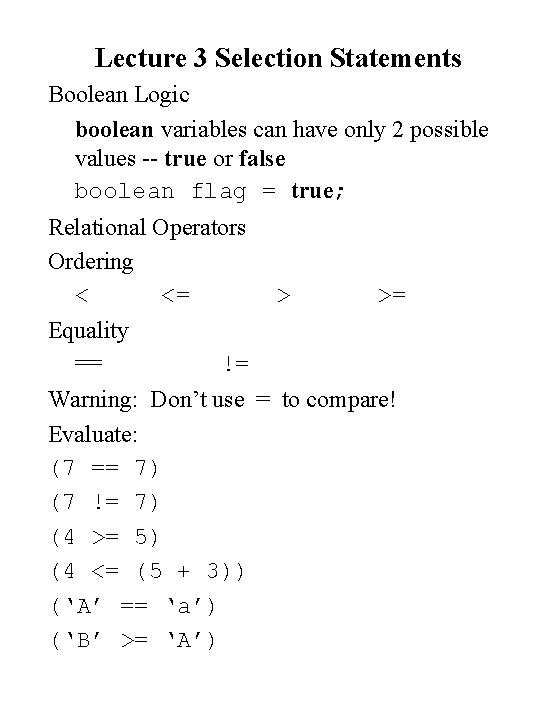Lecture 3 Selection Statements Boolean Logic boolean variables can have only 2 possible values -- true or false boolean flag = true; Relational Operators Ordering < <= Equality == > >= != Warning: Don’t use = to compare! Evaluate: (7 == 7) (7 != 7) (4 >= 5) (4 <= (5 + 3)) (‘A’ == ‘a’) (‘B’ >= ‘A’)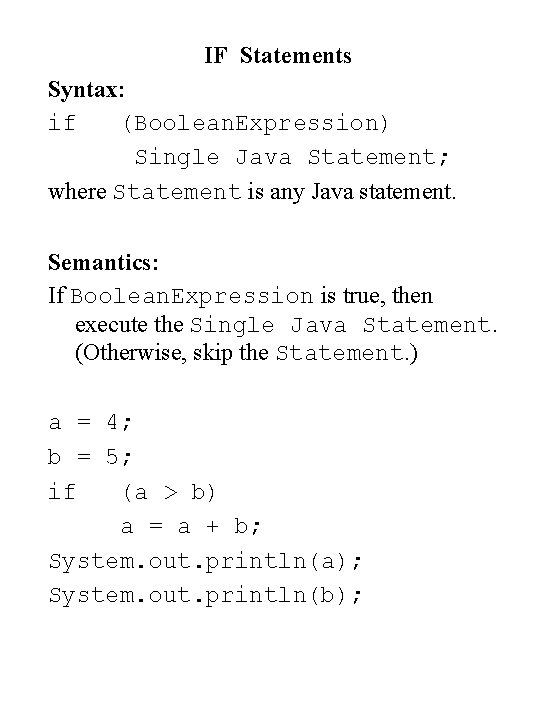IF Statements Syntax: if (Boolean. Expression) Single Java Statement; where Statement is any Java statement. Semantics: If Boolean. Expression is true, then execute the Single Java Statement. (Otherwise, skip the Statement. ) a = 4; b = 5; if (a > b) a = a + b; System. out. println(a); System. out. println(b);What if we want to execute several statements in then-part? Make a compound statement by using { and } if (num 1 > num 2){ num 1 = num 1 + 1; num 2 = num 2 - 1; } //if What is the output of these Java statements? (i) int x = 7; if(x > 8){ x = x * 2; System. out. println(x); } System. out. println(“Bye!”); (ii) int x = 7; if(x > 8) x = x * 2; System. out. println(x); System. out. println(“Bye!”);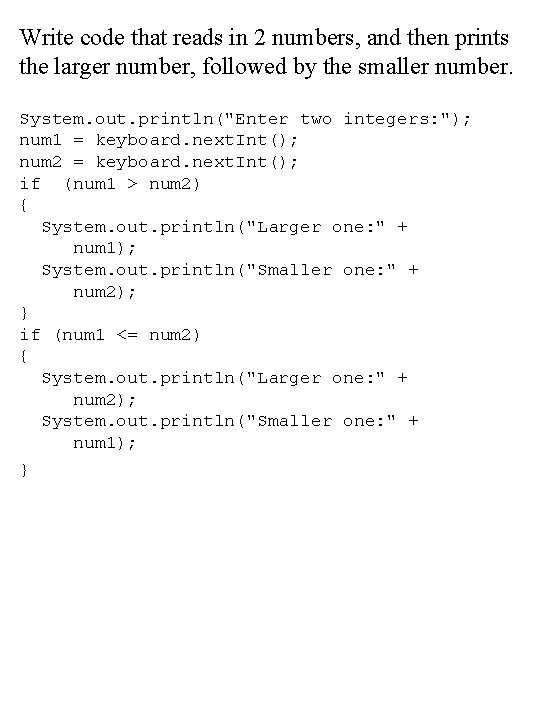Write code that reads in 2 numbers, and then prints the larger number, followed by the smaller number. System. out. println("Enter two integers: "); num 1 = keyboard. next. Int(); num 2 = keyboard. next. Int(); if (num 1 > num 2) { System. out. println("Larger one: " + num 1); System. out. println("Smaller one: " + num 2); } if (num 1 <= num 2) { System. out. println("Larger one: " + num 2); System. out. println("Smaller one: " + num 1); }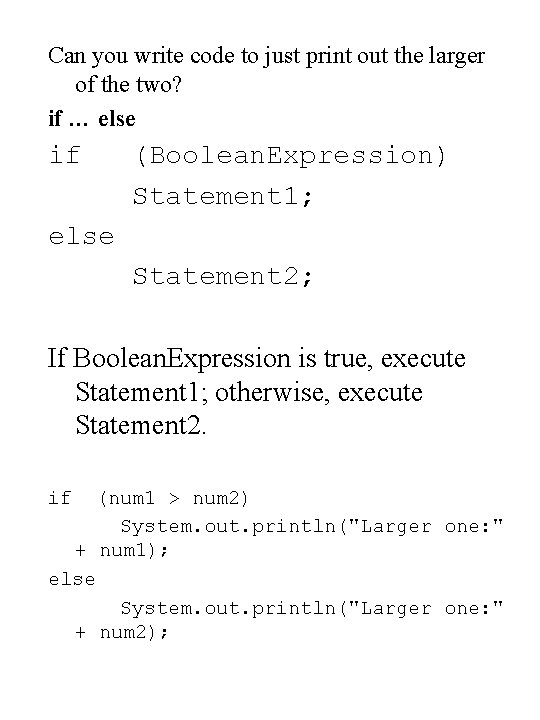Can you write code to just print out the larger of the two? if … else if (Boolean. Expression) Statement 1; else Statement 2; If Boolean. Expression is true, execute Statement 1; otherwise, execute Statement 2. if (num 1 > num 2) System. out. println("Larger one: " + num 1); else System. out. println("Larger one: " + num 2);Boolean Operators Truth Table Examples AND (&&) P Q True False (P && Q) True False OR (||) P True False Q True False (P || Q) True False P True False !(P) False True NOT (!)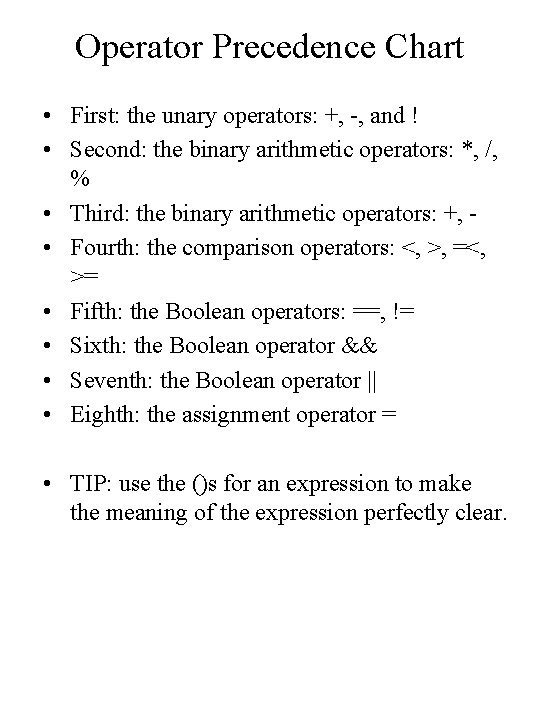Operator Precedence Chart • First: the unary operators: +, -, and ! • Second: the binary arithmetic operators: *, /, % • Third: the binary arithmetic operators: +, • Fourth: the comparison operators: <, >, =<, >= • Fifth: the Boolean operators: ==, != • Sixth: the Boolean operator && • Seventh: the Boolean operator || • Eighth: the assignment operator = • TIP: use the ()s for an expression to make the meaning of the expression perfectly clear.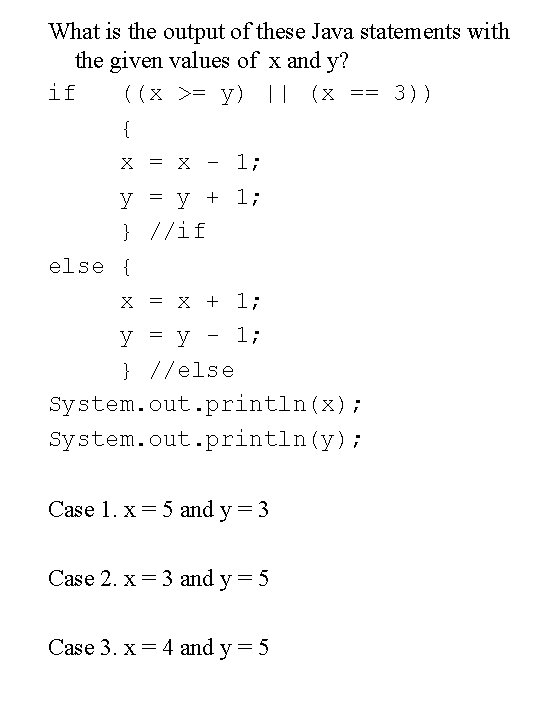What is the output of these Java statements with the given values of x and y? if ((x >= y) || (x == 3)) { x = x - 1; y = y + 1; } //if else { x = x + 1; y = y - 1; } //else System. out. println(x); System. out. println(y); Case 1. x = 5 and y = 3 Case 2. x = 3 and y = 5 Case 3. x = 4 and y = 5Nested if…else Statements if (cond 1) statement 1; else if (cond 2) statement 2; else if (cond 3) statement 3; else statement 4; if else (temp >= 100) ans = “Very Hot”; if (temp >= 90) ans = “Hot”; if (temp >= 70) ans = “Warm”; if (temp >= 50) ans = “Cool”; ans = “Cold”; • Write code that assigns a letter grade for a student, based on his/her score.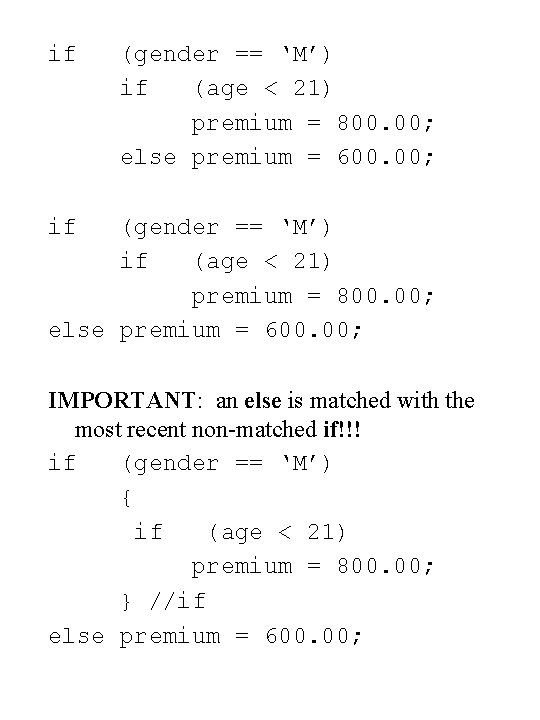if (gender == ‘M’) if (age < 21) premium = 800. 00; else premium = 600. 00; IMPORTANT: an else is matched with the most recent non-matched if!!! if (gender == ‘M’) { if (age < 21) premium = 800. 00; } //if else premium = 600. 00;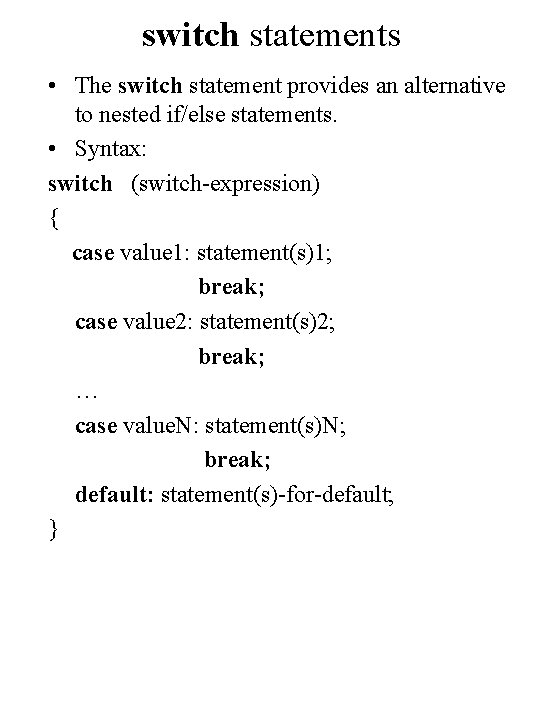switch statements • The switch statement provides an alternative to nested if/else statements. • Syntax: switch (switch-expression) { case value 1: statement(s)1; break; case value 2: statement(s)2; break; … case value. N: statement(s)N; break; default: statement(s)-for-default; }Rules of switch statement • The switch-expression must yield a value of int (byte, long) or char type since each case evaluates an == test. • The value 1, …, and value. N must have the same data type as the value of the switch-expression. They cannot contain variables in the expression. • The keyword break is optional. The break statement immediately ends the switch statement. • The case statements are checked in sequential order, but the order of the cases (including the default case) does not matter. However, it is good programming style to follow the logical sequence of the cases and place the default case at the end. • See pages 82 -83 for more detail.int score = ? ? ? ; switch (score/10) { case 10: case 9: letter. Grade = ‘A’; break; case 8: letter. Grade = ‘B’; break; case 7: letter. Grade = ‘C’; break; case 6: letter. Grade = ‘D’; break; default: letter. Grade = ‘F’; } //switch System. out. println(“Your letter grade is “ + letter. Grade); //Rewrite using nested if-else.//suppose score is out of 100 ans = score / 10; switch (ans) { case 0: case 1: case 2: case 3: case 4: case 5: System. out. println(“Grade is F”); break; case 6: System. out. println(“Grade is D”); break; case 7: System. out. println(“Grade is C”); break; case 8: System. out. println(“Grade is B”); break; case 9: case 10: System. out. println(“Grade is A”); default: System. out. println(“invalid grade!”); } // switch Write an equivalent nested if-else.